Examples

Chapter 4 Class 10 Quadratic Equations
Serial order wise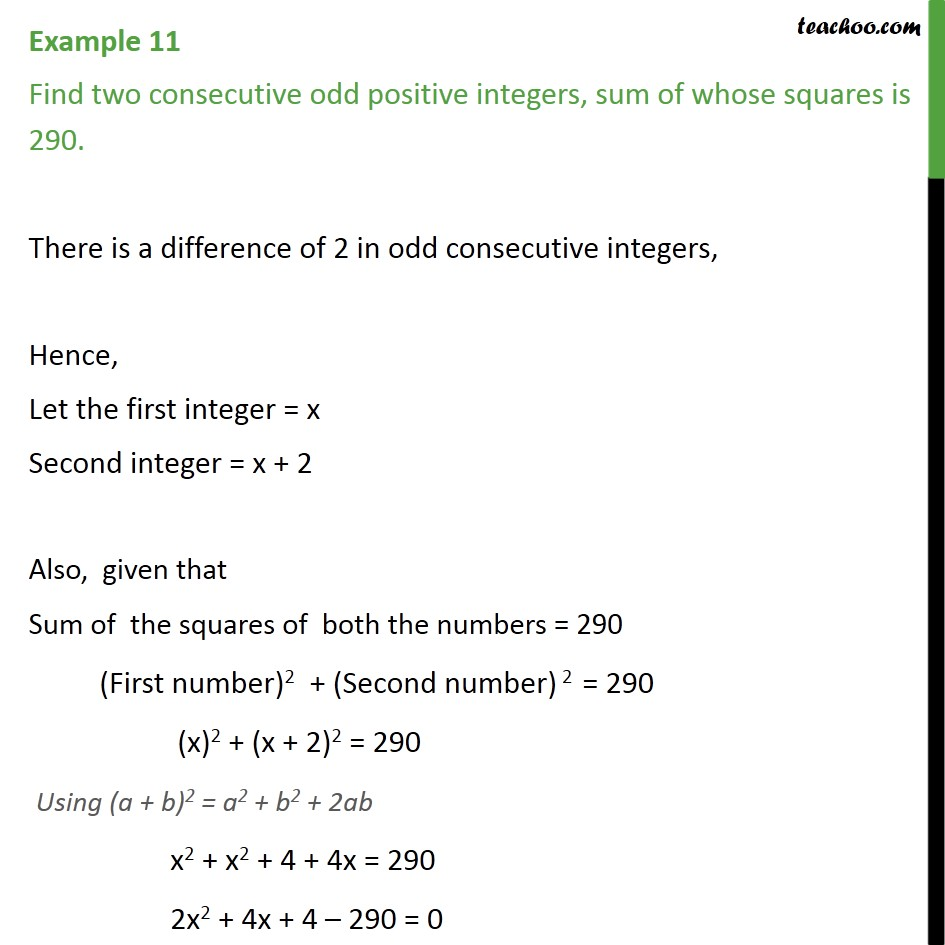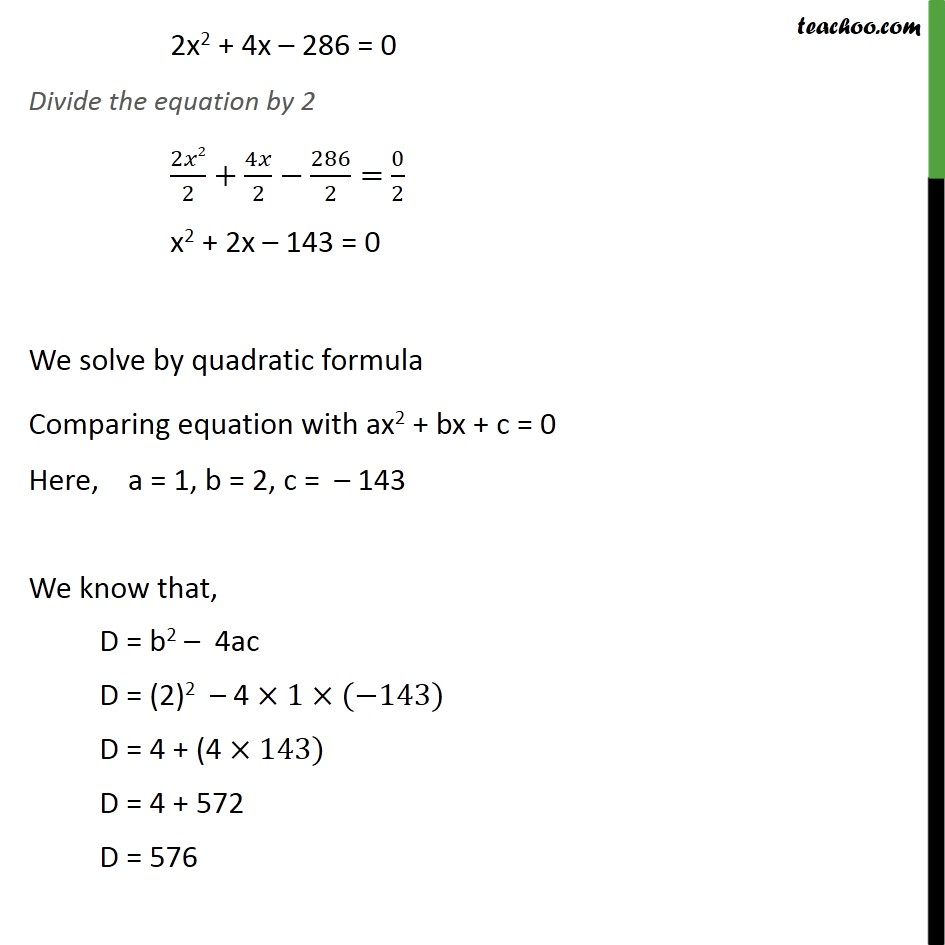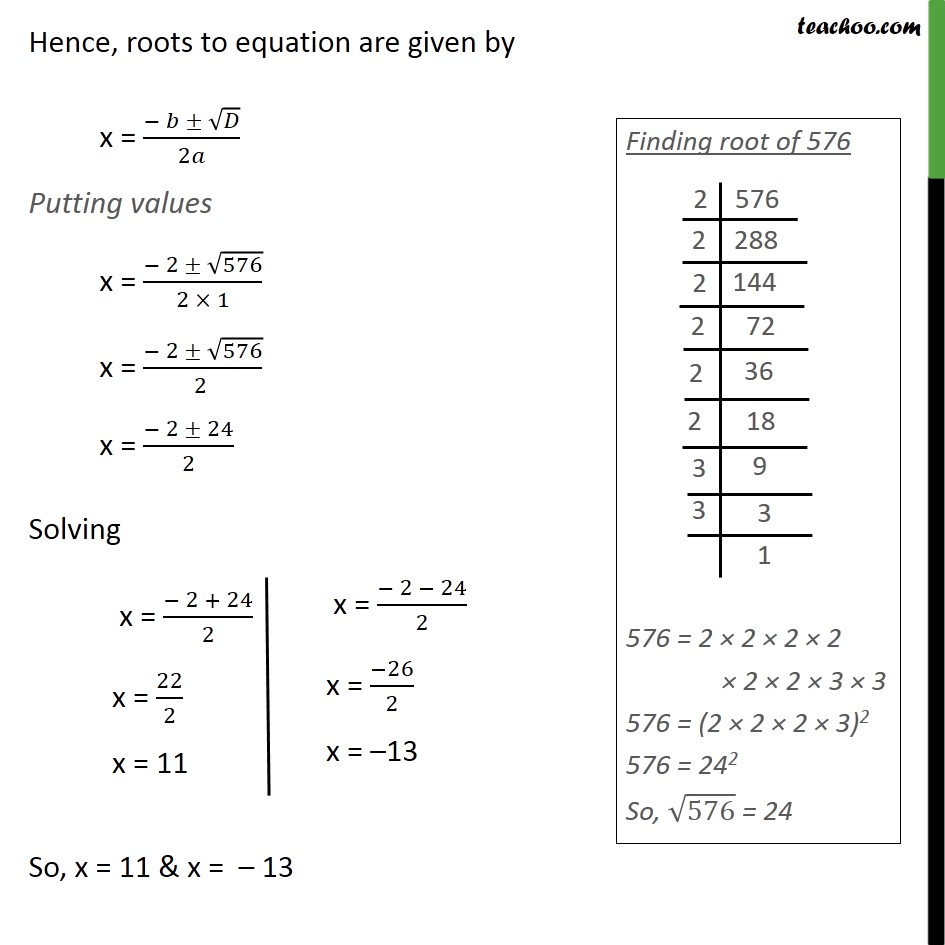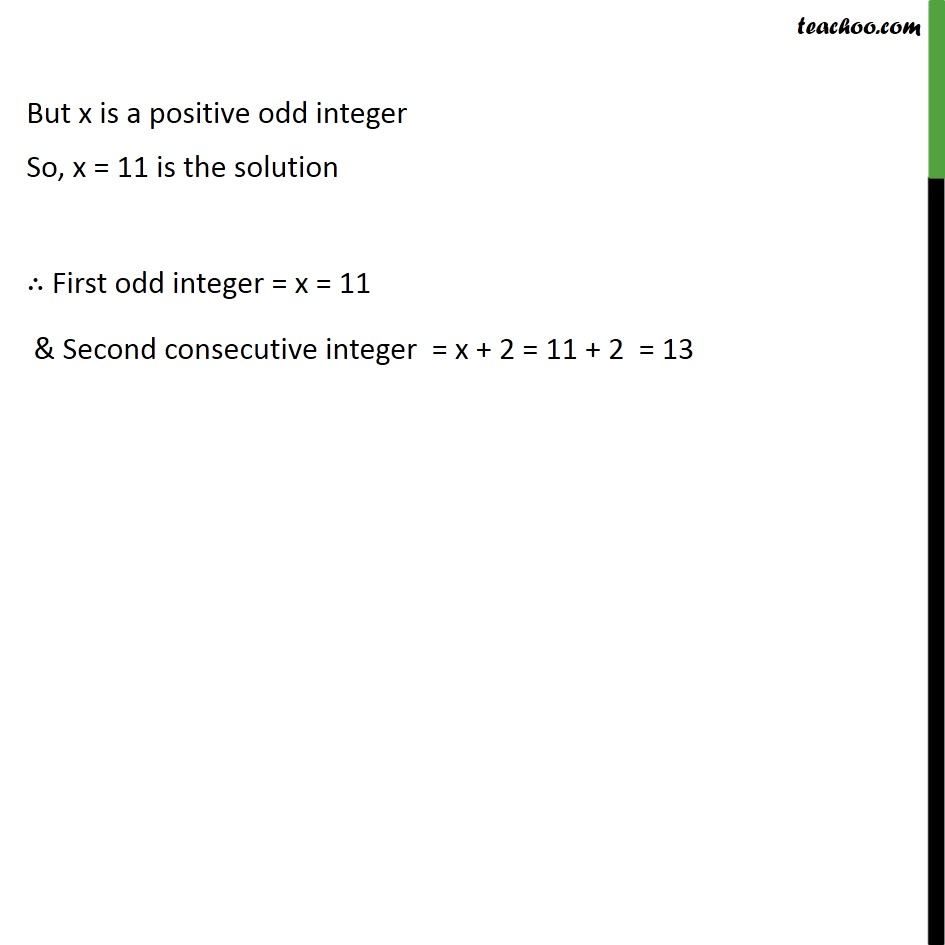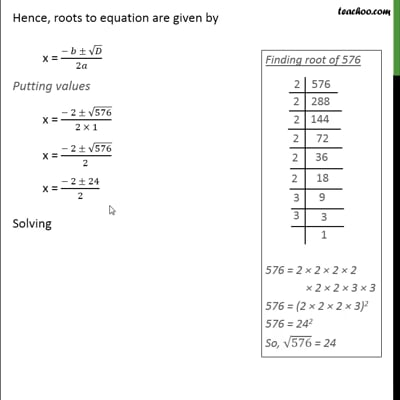This video is only available for Teachoo black users

Introducing your new favourite teacher - Teachoo Black, at only ₹83 per month

### Transcript

Example 11 Find two consecutive odd positive integers, sum of whose squares is 290. There is a difference of 2 in odd consecutive integers, Hence, Let the first integer = x Second integer = x + 2 Also, given that Sum of the squares of both the numbers = 290 (First number)2 + (Second number) 2 = 290 (x)2 + (x + 2)2 = 290 Using (a + b)2 = a2 + b2 + 2ab x2 + x2 + 4 + 4x = 290 2x2 + 4x + 4 290 = 0 2x2 + 4x 286 = 0 Divide the equation by 2 2 2/2+4 /2 286/2= 0/2 x2 + 2x 143 = 0 We solve by quadratic formula Comparing equation with ax2 + bx + c = 0 Here, a = 1, b = 2, c = 143 We know that, D = b2 4ac D = (2)2 4 1 ( 143) D = 4 + (4 143) D = 4 + 572 D = 576 Hence, roots to equation are given by x = ( )/2 Putting values x = ( 2 576)/(2 1) x = ( 2 576)/(2 ) x = ( 2 24)/2 Solving So, x = 11 & x = 13 But x is a positive odd integer So, x = 11 is the solution First odd integer = x = 11 & Second consecutive integer = x + 2 = 11 + 2 = 13